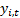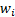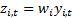/

### Blending Independent Components and Principal Components Analysis 3.2 Weighting schemas

Next page

3.2          Weighting schemas

There are certain types of problem where it is particularly desirable to ascribe different levels of ‘importance’ to different input signals according to their contribution to aggregate variability in the output signal ensemble.

A good example is portfolio risk measurement. We might characterise the return series coming from each individual stock as an output series and we might be seeking a parsimonious way of explaining the variability across the stock universe by assuming that there are a relatively modest number of underlying factors driving the behaviour of multiple stocks, together with some residual idiosyncratic risk factors applicable to each stock in isolation. The ultimate aim is to estimate some measure of the likely spread of returns that might arise from one particular portfolio (the actual portfolio chosen by the fund manager) relative to those on a benchmark portfolio (also drawn from the same universe but with the stocks differently weighted). A common proxy for spread here might be the standard deviation (or variance) of the relative return. However, this may not be a good proxy for fat-tailed distributions.

Commercial statistical factor risk models typically derive estimates of these underlying factor signals using principal components analysis. Suitably averaged across possible portfolios that might be chosen, the factors exhibiting the highest eigenvalues really are the ‘most important’ ones, because they explain the most variability across the universe as a whole, see Section 3.1. At least they do if variability and standard deviation/variance are equated as would the case for normally distributed random variables, but not necessarily for fat-tailed distributions. For these types of distributions, some refinement may be desirable, see Section 4.

Implicit in PCA is thus a weighting schema being applied to the different output signals. Suppose we multiply each individual output signalby a different weighting factor,, i.e. we now recast the problem as if the output signals were. This does not, in some sense, alter the available information we have to identify input signals. But what it does do is alter how much variability each given output series contributes to the total. It will therefore alter the coefficients defining the eigenvectors and which ones are deemed most important. Hence the results of PCA are not scale invariant in relation to individual stocks, since one of the implicit assumptions we are adopting is that a given quantum of output from any given signal has the same intrinsic ‘importance’ (in variability terms) as the same quantum of output from any other signal.

How does this compare with ICA? The projection pursuit method introduced earlier (and corresponding infomax and maximum likelihood ICA approaches) grade signal importance by reference to kurtosis, rather than by reference to contribution to overall variability. As we noted earlier, kurtosis is scale invariant. Thus ICA should identify ‘meaningful’ signals that influence the ensemble of output signals (if we are correct to ascribe ‘meaning’ to signals that appear to exhibit ‘independence’, ‘non-Normality’ or ‘lack of complexity’), but it will not necessarily preferentially select ones whose behaviours contribute significantly to the behaviour of the output signal ensemble.